# Check whether it is possible to join two points given on circle such that distance between them is k

Given two circles and a length K. Find whether we can join two points (one on perimeter of each circle), so that distance between the points is K. (Coordinates of both points need not to be an integer value).

Examples:

```Input: Circle-1 Center (0, 0) Radius = 5
Circle-2 Center (8, 3) Radius = 2
K = 3
Output: Yes
Maximum Distance: 15
Minimum Distance: 2
```

## Recommended: Please try your approach on {IDE} first, before moving on to the solution.

Approach:

1. We have to find the maximum and minimum distance possible between any two points on these circles, if K lies in this range than the answer is Yes otherwise we cannot find such a Line segment.
2. To find minimum and maximum distance:
• Case 1: When two circles does not intersect or just touches at one point.
In this scenario, the maximum distance would be distance between centers + Radius (circle 1) + Radius (circle 2). The minimum distance would be distance between centers – Radius(circle 1) – Radius (circle 2).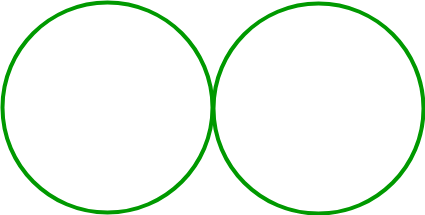• Case 2: When the two circles intersect at exactly two points.
In this scenario, the maximum distance would be distance between centers + Radius (circle 1) + Radius (circle 2). The minimum distance would be 0. (We have two points common on both the circles).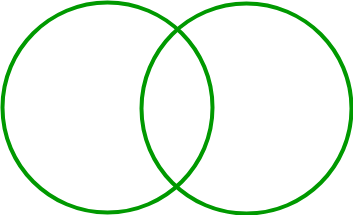• Case 3: When Circle 1 is completely inside Circle 2.
In this scenario, the maximum distance would be distance between centers + Radius (circle 1) + Radius (circle 2). The minimum distance would be Radius (Circle 2) – distance between centers – Radius (Circle 1)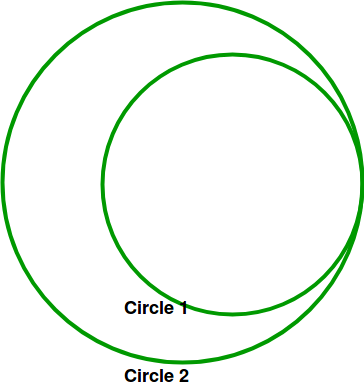• Case 4: When Circle 2 is completely inside Circle 1.
In this scenario, the maximum distance would be distance between centers + Radius (circle 1) + Radius (circle 2). The minimum distance would be Radius (Circle 1) – distance between centers – Radius (Circle 2)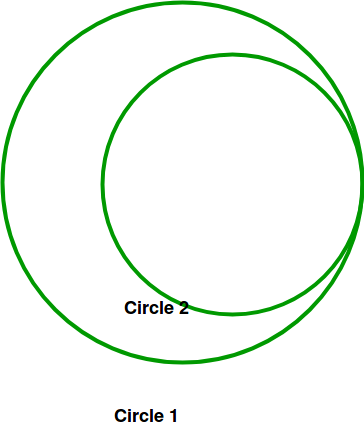• Case 5: When both Circles have same center
• Sub Case 1: Radius is also same. Both minimum distance and maximum distance are 0.
• Sub Case 2: Radius is different(R1<R2)
Maximum distance is R1+R2
Minimum distance is R2-R1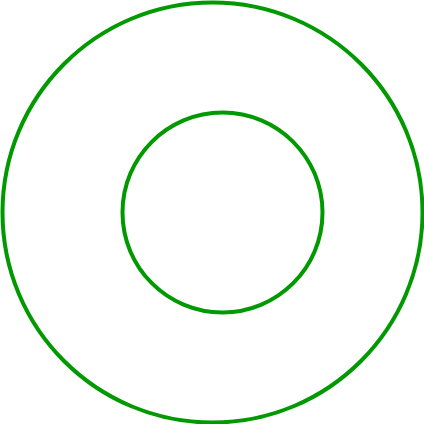Below is the implementation of above approach:

## C++

 `// C++ program to implement above approach ` `#include ` `#define ll long long int ` `using` `namespace` `std; ` ` `  `struct` `t { ` `    ``ll x, y, r; ` `}; ` `typedef` `struct` `t node; ` ` `  `// Return distance between the centers ` `long` `double` `dis(ll x1, ll y1, ll x2, ll y2) ` `{ ` `    ``return` `sqrt``((x1 - x2) * (x1 - x2)  ` `                ``+ (y1 - y2) * (y1 - y2)); ` `} ` ` `  `bool` `check(node c1, node c2, ``int` `k) ` `{ ` `    ``long` `double` `min = 0; ` `    ``long` `double` `max = 0; ` `    ``// Distance between centers ` `    ``long` `double` `de = dis(c1.x, c1.y, c2.x, c2.y); ` `    ``// Case 5 ` `    ``if` `(de == 0) { ` `        ``// SubCase 1 ` `        ``if` `(c1.r == c2.r) { ` `            ``min = 0; ` `            ``max = 0; ` `        ``} ` `        ``// Subcase 2 ` `        ``else` `{ ` `            ``if` `(c1.r - c2.r > 0) { ` `                ``min = c1.r - c2.r; ` `                ``max = min + 2 * c2.r; ` `            ``} ` `            ``else` `{ ` `                ``min = c2.r - c1.r; ` `                ``max = min + 2 * c1.r; ` `            ``} ` `        ``} ` `    ``} ` `    ``// Case 1 ` `    ``else` `if` `(de >= c1.r + c2.r) { ` `        ``min = de - c1.r - c2.r; ` `        ``max = de + c1.r + c2.r; ` `    ``} ` `    ``// Case 3 ` `    ``else` `if` `(de + c2.r < c1.r) { ` `        ``max = c2.r + c1.r + de; ` `        ``min = c1.r - de - c2.r; ` `    ``} ` `    ``// Case 4 ` `    ``else` `if` `(de + c1.r < c2.r) { ` ` `  `        ``max = c2.r + c1.r + de; ` `        ``min = c2.r - de - c1.r; ` `    ``} ` `    ``// Case 2 ` `    ``else` `if` `((de + c2.r >= c1.r) || (de + c1.r >= c2.r)) { ` `        ``max = c2.r + c1.r + de; ` `        ``min = 0; ` `    ``} ` `    ``// Since value of k will always be an integer ` `    ``ll temin = (ll)(``ceil``(min)); ` `    ``ll re = (ll)max; ` `    ``if` `(k >= temin && k <= re) ` `        ``return` `true``; ` `    ``return` `false``; ` `} ` ` `  `// Driver Code ` `int` `main() ` `{ ` `    ``node circle1, circle2; ` `    ``int` `k = 3; ` `    ``circle1.x = 0; ` `    ``circle1.y = 0; ` `    ``circle1.r = 5; ` `    ``circle2.x = 8; ` `    ``circle2.y = 3; ` `    ``circle2.r = 2; ` `    ``if` `(check(circle1, circle2, k)) ` `        ``cout << ``"YES"` `<< endl; ` `    ``else` `        ``cout << ``"NO"` `<< endl; ` `} `

## Java

 `// Java program to implement above approach ` `class` `GFG  ` `{ ` ` `  `    ``static` `class` `node  ` `    ``{ ` `        ``long` `x, y, r; ` `    ``}; ` ` `  `    ``// Return distance between the centers ` `    ``static` `long` `dis(``long` `x1, ``long` `y1, ``long` `x2, ``long` `y2)  ` `    ``{ ` `        ``return` `(``long``) Math.sqrt((x1 - x2) * (x1 - x2) ` `                ``+ (y1 - y2) * (y1 - y2)); ` `    ``} ` ` `  `    ``static` `boolean` `check(node c1, node c2, ``int` `k) ` `    ``{ ` `        ``long` `min = ``0``; ` `        ``long` `max = ``0``; ` `         `  `        ``// Distance between centers ` `        ``long` `de = dis(c1.x, c1.y, c2.x, c2.y); ` `         `  `        ``// Case 5 ` `        ``if` `(de == ``0``)  ` `        ``{ ` `            ``// SubCase 1 ` `            ``if` `(c1.r == c2.r) ` `            ``{ ` `                ``min = ``0``; ` `                ``max = ``0``; ` `            ``}  ` `            ``// Subcase 2 ` `            ``else` `if` `(c1.r - c2.r > ``0``)  ` `            ``{ ` `                ``min = c1.r - c2.r; ` `                ``max = min + ``2` `* c2.r; ` `            ``}  ` `            ``else` `            ``{ ` `                ``min = c2.r - c1.r; ` `                ``max = min + ``2` `* c1.r; ` `            ``} ` `        ``}  ` `         `  `        ``// Case 1 ` `        ``else` `if` `(de >= c1.r + c2.r)  ` `        ``{ ` `            ``min = de - c1.r - c2.r; ` `            ``max = de + c1.r + c2.r; ` `        ``}  ` `        ``// Case 3 ` `        ``else` `if` `(de + c2.r < c1.r) ` `        ``{ ` `            ``max = c2.r + c1.r + de; ` `            ``min = c1.r - de - c2.r; ` `        ``}  ` `        ``// Case 4 ` `        ``else` `if` `(de + c1.r < c2.r)  ` `        ``{ ` ` `  `            ``max = c2.r + c1.r + de; ` `            ``min = c2.r - de - c1.r; ` `        ``} ` `        ``// Case 2 ` `        ``else` `if` `((de + c2.r >= c1.r) || (de + c1.r >= c2.r)) ` `        ``{ ` `            ``max = c2.r + c1.r + de; ` `            ``min = ``0``; ` `        ``} ` `         `  `        ``// Since value of k will always be an integer ` `        ``long` `temin = (``long``) (Math.ceil(min)); ` `        ``long` `re = (``long``) max; ` `        ``if` `(k >= temin && k <= re) ` `        ``{ ` `            ``return` `true``; ` `        ``} ` `        ``return` `false``; ` `    ``} ` ` `  `    ``// Driver Code ` `    ``public` `static` `void` `main(String[] args)  ` `    ``{ ` `        ``node circle1 = ``new` `node(); ` `        ``node circle2 = ``new` `node(); ` `        ``int` `k = ``3``; ` `        ``circle1.x = ``0``; ` `        ``circle1.y = ``0``; ` `        ``circle1.r = ``5``; ` `        ``circle2.x = ``8``; ` `        ``circle2.y = ``3``; ` `        ``circle2.r = ``2``; ` `        ``if` `(check(circle1, circle2, k)) ` `        ``{ ` `            ``System.out.println(``"Yes"``); ` `        ``}  ` `        ``else` `        ``{ ` `            ``System.out.println(``"No"``); ` `        ``} ` `    ``} ` `} ` ` `  `// This code is contributed by Princi Singh `

## Python

 `# Python3 program to implement above approach ` `from` `math ``import` `sqrt,ceil,floor ` ` `  `# Return distance between the centers ` `def` `dis(x1, y1, x2, y2): ` `    ``return` `sqrt((x1 ``-` `x2) ``*` `(x1 ``-` `x2) ``+`  `                ``(y1 ``-` `y2) ``*` `(y1 ``-` `y2)) ` ` `  `def` `check(c1, c2, k): ` `    ``min` `=` `0` `    ``max` `=` `0` `     `  `    ``# Distance between centers ` `    ``de ``=` `dis(c1[``0``], c1[``1``], c2[``0``], c2[``1``]) ` `     `  `    ``# Case 5 ` `    ``if` `(de ``=``=` `0``): ` `         `  `        ``# SubCase 1 ` `        ``if` `(c1[``2``] ``=``=` `c2[``2``]): ` `            ``min` `=` `0` `            ``max` `=` `0` `     `  `        ``# Subcase 2 ` `        ``else``: ` `            ``if` `(c1[``2``] ``-` `c2[``2``] > ``0``): ` `                ``min` `=` `c1[``2``] ``-` `c2[``2``] ` `                ``max` `=` `min` `+` `2` `*` `c2[``2``] ` ` `  `            ``else``: ` `                ``min` `=` `c2[``2``] ``-` `c1[``2``] ` `                ``max` `=` `min` `+` `2` `*` `c1[``2``] ` ` `  `    ``# Case 1 ` `    ``elif` `(de >``=` `c1[``2``] ``+` `c2[``2``]): ` `        ``min` `=` `de ``-` `c1[``2``] ``-` `c2[``2``] ` `        ``max` `=` `de ``+` `c1[``2``] ``+` `c2[``2``] ` `     `  `    ``# Case 3 ` `    ``elif` `(de ``+` `c2[``2``] < c1[``2``]): ` `        ``max` `=` `c2[``2``] ``+` `c1[``2``] ``+` `de ` `        ``min` `=` `c1[``2``] ``-` `de ``-` `c2[``2``] ` `     `  `    ``# Case 4 ` `    ``elif` `(de ``+` `c1[``2``] < c2[``2``]): ` ` `  `        ``max` `=` `c2[``2``] ``+` `c1[``2``] ``+` `de ` `        ``min` `=` `c2[``2``] ``-` `de ``-` `c1[``2``] ` `     `  `    ``# Case 2 ` `    ``elif` `((de ``+` `c2[``2``] >``=` `c1[``2``]) ``or` `(de ``+` `c1[``2``] >``=` `c2[``2``])): ` `        ``max` `=` `c2[``2``] ``+` `c1[``2``] ``+` `de ` `        ``min` `=` `0` ` `  `    ``# Since value of k wialways be an integer ` `    ``temin ``=` `ceil(``min``) ` `    ``re ``=` `max` `    ``if` `(k >``=` `temin ``and` `k <``=` `re): ` `        ``return` `True` `    ``return` `False` ` `  `# Driver Code ` `circle1 ``=` `[``0``, ``0``, ``5``] ` `circle2 ``=` `[``8``, ``3``, ``2``] ` `k ``=` `3` ` `  `if` `(check(circle1, circle2, k)): ` `    ``print``(``"YES"``) ` `else``: ` `    ``print``(``"NO"` `) ` `     `  `# This code is contributed by mohit kumar 29 `

## C#

 `// C# program to implement above approach  ` `using` `System; ` `     `  `class` `GFG  ` `{ ` ` `  `    ``public` `class` `node  ` `    ``{ ` `        ``public` `long` `x, y, r; ` `    ``}; ` ` `  `    ``// Return distance between the centers ` `    ``static` `long` `dis(``long` `x1, ``long` `y1, ``long` `x2, ``long` `y2)  ` `    ``{ ` `        ``return` `(``long``) Math.Sqrt((x1 - x2) * (x1 - x2) ` `                ``+ (y1 - y2) * (y1 - y2)); ` `    ``} ` ` `  `    ``static` `Boolean check(node c1, node c2, ``int` `k) ` `    ``{ ` `        ``long` `min = 0; ` `        ``long` `max = 0; ` `         `  `        ``// Distance between centers ` `        ``long` `de = dis(c1.x, c1.y, c2.x, c2.y); ` `         `  `        ``// Case 5 ` `        ``if` `(de == 0)  ` `        ``{ ` `            ``// SubCase 1 ` `            ``if` `(c1.r == c2.r) ` `            ``{ ` `                ``min = 0; ` `                ``max = 0; ` `            ``}  ` `            ``// Subcase 2 ` `            ``else` `if` `(c1.r - c2.r > 0)  ` `            ``{ ` `                ``min = c1.r - c2.r; ` `                ``max = min + 2 * c2.r; ` `            ``}  ` `            ``else` `            ``{ ` `                ``min = c2.r - c1.r; ` `                ``max = min + 2 * c1.r; ` `            ``} ` `        ``}  ` `         `  `        ``// Case 1 ` `        ``else` `if` `(de >= c1.r + c2.r)  ` `        ``{ ` `            ``min = de - c1.r - c2.r; ` `            ``max = de + c1.r + c2.r; ` `        ``}  ` `        ``// Case 3 ` `        ``else` `if` `(de + c2.r < c1.r) ` `        ``{ ` `            ``max = c2.r + c1.r + de; ` `            ``min = c1.r - de - c2.r; ` `        ``}  ` `        ``// Case 4 ` `        ``else` `if` `(de + c1.r < c2.r)  ` `        ``{ ` ` `  `            ``max = c2.r + c1.r + de; ` `            ``min = c2.r - de - c1.r; ` `        ``} ` `        ``// Case 2 ` `        ``else` `if` `((de + c2.r >= c1.r) || (de + c1.r >= c2.r)) ` `        ``{ ` `            ``max = c2.r + c1.r + de; ` `            ``min = 0; ` `        ``} ` `         `  `        ``// Since value of k will always be an integer ` `        ``long` `temin = (``long``) (Math.Ceiling((``double``)min)); ` `        ``long` `re = (``long``) max; ` `        ``if` `(k >= temin && k <= re) ` `        ``{ ` `            ``return` `true``; ` `        ``} ` `        ``return` `false``; ` `    ``} ` ` `  `    ``// Driver Code ` `    ``public` `static` `void` `Main(String[] args)  ` `    ``{ ` `        ``node circle1 = ``new` `node(); ` `        ``node circle2 = ``new` `node(); ` `        ``int` `k = 3; ` `        ``circle1.x = 0; ` `        ``circle1.y = 0; ` `        ``circle1.r = 5; ` `        ``circle2.x = 8; ` `        ``circle2.y = 3; ` `        ``circle2.r = 2; ` `        ``if` `(check(circle1, circle2, k)) ` `        ``{ ` `            ``Console.WriteLine(``"Yes"``); ` `        ``}  ` `        ``else` `        ``{ ` `            ``Console.WriteLine(``"No"``); ` `        ``} ` `    ``} ` `} ` ` `  `// This code contributed by Rajput-Ji `

Output:

```YES
```My Personal Notes arrow_drop_upCheck out this Author's contributed articles.

If you like GeeksforGeeks and would like to contribute, you can also write an article using contribute.geeksforgeeks.org or mail your article to contribute@geeksforgeeks.org. See your article appearing on the GeeksforGeeks main page and help other Geeks.

Please Improve this article if you find anything incorrect by clicking on the "Improve Article" button below.